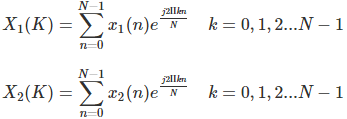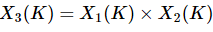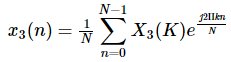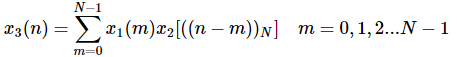Courses

Circular Convolution - Discrete Fourier Transform Notes | Study Signals and Systems - Electrical Engineering (EE)

Electrical Engineering (EE): Circular Convolution - Discrete Fourier Transform Notes | Study Signals and Systems - Electrical Engineering (EE)

The document Circular Convolution - Discrete Fourier Transform Notes | Study Signals and Systems - Electrical Engineering (EE) is a part of the Electrical Engineering (EE) Course Signals and Systems.
All you need of Electrical Engineering (EE) at this link: Electrical Engineering (EE)

Let us take two finite duration sequences x1(n) and x2(n), having integer length as N. Their DFTs are X1(K) and X2(K) respectively, which is shown below −Now, we will try to find the DFT of another sequence x3(n), which is given as X3(K)By taking the IDFT of the above we getAfter solving the above equation, finally, we getComparison pointsLinear ConvolutionCircular Convolution
ShiftingLinear shiftingCircular shifting
Samples in the convolution resultN1+N2−1Max(N1,N2)
Finding response of a filterPossiblePossible with zero padding

Methods of Circular Convolution

Generally, there are two methods, which are adopted to perform circular convolution and they are −

• Concentric circle method,
• Matrix multiplication method.

Concentric Circle Method

Let x1(n) and x2(n) be two given sequences. The steps followed for circular convolution of x1(n) and x2(n) are

• Take two concentric circles. Plot N samples of x1(n) on the circumference of the outer circle (maintaining equal distance successive points) in anti-clockwise direction.

• For plotting x2(n), plot N samples of x2(n) in clockwise direction on the inner circle, starting sample placed at the same point as 0thsample of x1(n)

• Multiply corresponding samples on the two circles and add them to get output.

• Rotate the inner circle anti-clockwise with one sample at a time.

Matrix Multiplication Method

Matrix method represents the two given sequence x1(n) and x2(n) in matrix form.

• One of the given sequences is repeated via circular shift of one sample at a time to form a N X N matrix.

• The other sequence is represented as column matrix.

• The multiplication of two matrices give the result of circular convolution.

The document Circular Convolution - Discrete Fourier Transform Notes | Study Signals and Systems - Electrical Engineering (EE) is a part of the Electrical Engineering (EE) Course Signals and Systems.
All you need of Electrical Engineering (EE) at this link: Electrical Engineering (EE)Use Code STAYHOME200 and get INR 200 additional OFF Use Coupon Code

Top Courses for Electrical Engineering (EE)Signals and Systems

32 videos|74 docs|56 tests

Top Courses for Electrical Engineering (EE)Track your progress, build streaks, highlight & save important lessons and more!

,

,

,

,

,

,

,

,

,

,

,

,

,

,

,

,

,

,

,

,

,

;